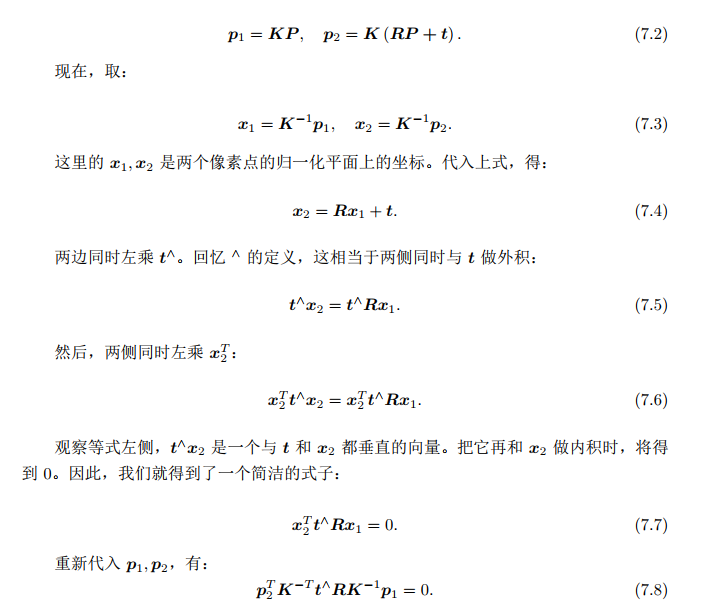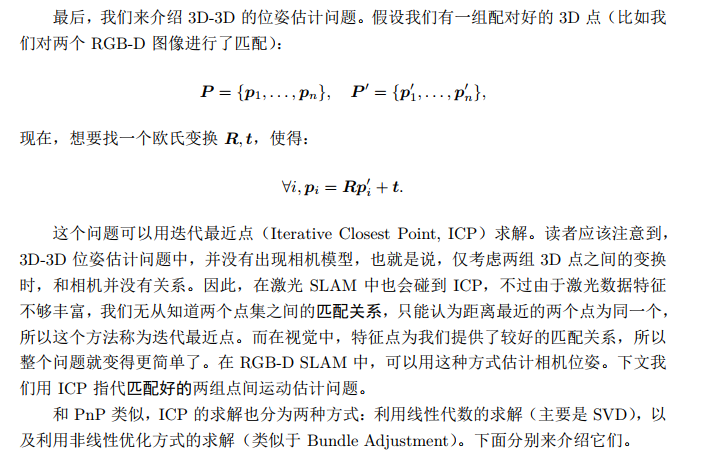• 学习SLAM-ICP原理及应用,内含自动驾驶学习资料
• ## 二维点云ICP原理推导

千次阅读 多人点赞 2020-05-28 00:01:25
二维点云ICP原理推导 描述 ICP是迭代就近点算法，大部分的实现代码都是基于PCL点云库的，也就是三维点云的匹配 实际上，二维点云数据也算是常见的数据类型，比如移动机器人经常使用的单线雷达。本文就是二维点云ICP...
二维点云ICP原理推导
描述
ICP是迭代就近点算法，大部分的实现代码都是基于PCL点云库的，也就是三维点云的匹配
实际上，二维点云数据也算是常见的数据类型，比如移动机器人经常使用的单线雷达。本文就是二维点云ICP的原理推导
算法原理
二维点云数据说明
先说明单线激光雷达数据类型
$data=\left[ \begin{array}{c} r_{i} \\ \theta _{i} \end{array} \right]$
r和θ代表每一束激光的距离和扫描角度，i代表雷达扫描一圈的每束激光。因此每帧点云数据根据极坐标变换后就是
$A=\; \left[ \begin{array}{c} x_{i} \\ y_{i} \end{array} \right]\; =\; \left[ \begin{array}{c} r_{i}\cdot \cos \theta \\ r_{i}\cdot \sin \theta \end{array} \right]$
现在假设我们有两帧点云A与B，我们把 A 称为标准点云，把 B 称为源点云。我们的需求是把点云 B 经过矩阵变换到点云 A，需要注意的是点云 A 和点云 B 是在同一坐标系下。
矩阵变换计算
在同一坐标系下，将某一片点云变换到另外一片点云，需要矩阵变换的知识。
假设我们需要点云在x方向平移Δx， y方向平移Δy， 点云整体旋转θ弧度
旋转矩阵R如下
$R=\left[ \begin{array}{cc} \cos \theta & \sin \theta \\ -\sin \theta & \cos \theta \end{array} \right]$
平移向量t如下
$t\; =\; \left[ \begin{array}{c} \Delta x \\ \Delta y \end{array} \right]$
则变换后的点云$A'$为
$A'=R\cdot A+t$
ICP算法核心
ICP算法很简单，简单来说就是对b点云不停进行矩阵变换，把它变换到和a很接近就停止。专业的说，分为如下几步

点云归一化
找到两片点云中的对应点对
根据对应点对的信息，计算得到这次的变换矩阵
变换点云
计算当前的损失
是否决定继续迭代。如果损失小于设定阈值或者达到最大迭代数停止，否则返回执行第1步

1. 点云归一
点云归一化只是我的一个说法，实际并不完全等同传统的归一化操作。
这里做的操作是，取点云的中心（或者有权重时可以取重心，或者其他合理的点也是可以的）
$A_{center}\; =\frac{1}{n}\sum_{i=1}^{n}{A_{i}}$
$B_{center}\; =\frac{1}{n}\sum_{i=1}^{n}{B_{i}}$
这里$A_{center}$和$B_{center}$实际就是点云A和点云B中的平均值。$A_{i}$和$B_{i}$是点云中的第i个点。接下来将每个点都减去这个平均值。
$a_{i}=\; A_{i}-A_{center}$
$b_{i}=\; B_{i}-B_{center}$
接下来的一部分操作是要使用归一化后的点集 $a$ 和  $b$，有一部分是要使用原来的点云 $A$ 和  $B$ 的，请注意区分
2. 确定对应点对
确定对应点对这是一个比较有意思的话题。什么是对应点对？
正确的理解就是，点云A和点云B的这两个点，可能原来就是一个点。但实际上，当A和B还没有经过ICP时，往往这个关系是模糊的，没有人能够说出哪两个点是对应的。那该怎么办呢？好啦，ICP的核心来啦。
ICP算法的对应点对，计算欧式距离最近的被认为是对应点对，也就是ICP的C，closet
具体实施上可以这样做，经过归一化后的点集 $a$ 和  $b$中，$a_{i}$ 的对应点 $b_{j}$ 是
$\left\{ a_{i},b_{j} \right\}\; =arg\min _{j}\left\{ \sqrt{\left( a_{i}^{x}-b_{j}^{x} \right)^{2}+\left( a_{i}^{y}-b_{j}^{y} \right)^{2}} \right\}$
在PCL库的ICP算法过程中，有一个很重要的步骤就是对应关系去除。原因是数据是有噪声的，因此要去除对配准有影响的错误点对。
二维点云的数据量是小的，那么请你结合自己的需求来完成去噪这一步。这一步是必要的。
3. 变换矩阵计算
先明确到了这一步我们拥有的数据和要得到的东西。
我们有的是很多对应的点对，我们要求的是一个变换矩阵，使用这个矩阵完成变换后，这些对应的点对的距离和是最小的（意思就是说，这个矩阵至少是当前最优的，使用这个矩阵相比于其他任何变换矩阵，能让牛郎们和织女们的距离和最小）。那么我们就来进行公式推导吧。（数学老师说的没错，数学真的很重要）
设置对应点对的距离和函数为$D(R,t)$
$D\left( R,t \right)=\frac{1}{n}\sum_{i=1}^{n}{\left| a_{i}-R\cdot b_{i} \right|^{2}}$
拆开平方项的结果就是如下
$D\left( R,t \right)=\frac{1}{n}\left( \sum_{i=1}^{n}{\left| a_{i} \right|^{2}}+\sum_{i=1}^{n}{\left| R\cdot b_{i} \right|^{2}}-2\sum_{i=1}^{n}{\left| a_{i}Rb_{i} \right|} \right)$
由于前两项是定值（${\left| R\cdot b_{i} \right|^{2}}$的值就是$(b_i^x)^2+(b_i^y)^2$的值，是不会随着R的变化发生改变）
因此，误差函数实际上可以认为是$E\left( R,t \right)$
$E\left( R,t \right) =\sum_{i=1}^{n}{\left| a_{i}Rb_{i} \right|}$
误差函数值$E\left( R,t \right)$越大，总的距离和越小。
在这里我好好推一下公式，说明一下这是怎么得到的吧
距离和函数为$D(R,t)$其实就是对所有的对应点对距离来求和。直接写成矩阵形式会更好理解
$D\left( R,t \right)\; =\frac{1}{n}\sum_{i=1}^{n}{\left| \left[ \begin{array}{c} a_{i}^{x} \\ a_{i}^{y} \end{array} \right]-\left[ \begin{array}{cc} \cos \theta & \sin \theta \\ -\sin \theta & \cos \theta \end{array} \right]\cdot \left[ \begin{array}{c} b_{i}^{x} \\ b_{i}^{y} \end{array} \right] \right|^{2}}$
$=\frac{1}{n}\sum_{i=1}^{n}{\left| \left[ \begin{array}{c} a_{i}^{x}-\cos \theta \cdot b_{i}^{x}-\sin \theta \cdot b_{i}^{y} \\ a_{i}^{y}+\sin \theta \cdot b_{i}^{x}-\cos \theta \cdot b_{i}^{y} \end{array} \right] \right|^{2}}$
$=\frac{1}{n}\sum_{i=1}^{n}{\left[ \left( a_{i}^{x}-\cos \theta \cdot b_{i}^{x}-\sin \theta \cdot b_{i}^{y} \right)^{2}+\left( a_{i}^{y}+\sin \theta \cdot b_{i}^{x}-\cos \theta \cdot b_{i}^{y} \right)^{2} \right]}$
这步推导我真的不写详细在这里，因为已经真的很简单了，推到下一步如下
$=\frac{1}{n}\sum_{i=1}^{n}{\left\{ \left( a_{i}^{x} \right)^{2}+\left( a_{i}^{y} \right)^{2}+\left( b_{i}^{x} \right)^{2}+\left( b_{i}^{y} \right)^{2}-2\left[ \cos \theta \left( a_{i}^{x}\cdot b_{i}^{x}+a_{i}^{y}\cdot b_{i}^{y} \right)+\sin \theta \left( a_{i}^{x}\cdot b_{i}^{y}-a_{i}^{y}\cdot b_{i}^{x} \right) \right] \right\}}$
所以推导出误差函数如下
$E\left( R,t \right)=\frac{1}{n}\sum_{i=1}^{n}{\left\{ \left[ \cos \theta \left( a_{i}^{x}\cdot b_{i}^{x}+a_{i}^{y}\cdot b_{i}^{y} \right)+\sin \theta \left( a_{i}^{x}\cdot b_{i}^{y}-a_{i}^{y}\cdot b_{i}^{x} \right) \right] \right\}}$
这里的变量只有 $θ$ ，对误差函数求导，就能得出它的极大值
$\frac{\sigma E\left( R,t \right)}{\sigma \theta }=\frac{1}{n}\sum_{i=1}^{n}{\left\{ \left[ -\sin \theta \left( a_{i}^{x}\cdot b_{i}^{x}+a_{i}^{y}\cdot b_{i}^{y} \right)+\cos \theta \left( a_{i}^{x}\cdot b_{i}^{y}-a_{i}^{y}\cdot b_{i}^{x} \right) \right] \right\}}=0$
因此进一步推导
$\tan \theta =\frac{\sum_{i=1}^{n}{\left( a_{i}^{x}\cdot b_{i}^{y}-a_{i}^{y}\cdot b_{i}^{x} \right)}}{\sum_{i=1}^{n}{\left( a_{i}^{x}\cdot b_{i}^{x}+a_{i}^{y}\cdot b_{i}^{y} \right)}}$
旋转弧度$θ$的计算如下
$\theta =\arctan \left( \frac{\sum_{i=1}^{n}{\left( a_{i}^{x}\cdot b_{i}^{y}-a_{i}^{y}\cdot b_{i}^{x} \right)}}{\sum_{i=1}^{n}{\left( a_{i}^{x}\cdot b_{i}^{x}+a_{i}^{y}\cdot b_{i}^{y} \right)}} \right)$
旋转矩阵 $R$ 因此也能得到
平移向量$t$的计算方式如下
$t\; =\; \left[ \begin{array}{c} \Delta x \\ \Delta y \end{array} \right]=\left[ \begin{array}{c} A_{center}^{x} \\ A_{center}^{y} \end{array} \right]-R\left[ \begin{array}{c} B_{center}^{x} \\ B_{center}^{y} \end{array} \right]$
解释一下也很简单，本来是可以A中心减B中心的，但是刚才已经证明了经过旋转矩阵 $R$ 会令点对距离和更小，因此B的中心点位置也就同时经历矩阵 $R$ 的变换
4. 变换点云
第三步我们已经求出了旋转矩阵 $R$ 和平移向量 $t$，接下来就是将原点云进行矩阵变换了。注意，是原来的点云 $A$ 和点云 $B$ ，而不是归一化后的点云 $a$ 和点云 $b$。为什么？你的操作对象一直是点云 $A$ 和点云 $B$ ，归一化后的 $a$ 和 $b$ 只是你需要的中间变量而已
对源点云 $B$ 做变换，得到新的点云 $B'$
$B'\; =\; R\cdot B+t$
5. 计算损失
将最新变换出的矩阵 $B'$，与你的目标点云 $A$ 进行计算。可以自行设计属于自己的损失函数，来表示两片点云究竟匹配的怎么样。
我在这里设计的损失函数仍然是对应点对（最近点）的距离之和
$Loss= D\left( R,t \right)=\frac{1}{n}\sum_{i=1}^{n}{\left| A_{i}- B^’_{i} \right|^{2}}$
6. 是否继续迭代
接下来就要看这个计算出的 $Loss$ 值能否满足你的要求了。
迭代终止条件的设计其实比较自由。但我的经验告诉我，比较常见的是以下几种

$Loss$ < $Loss_{min}$ : 当前迭代得到的损失值小于了设定的最小阈值了
$iteration$ < $iteration_{max}$  : 迭代次数超过了最大迭代次数
$ΔLoss$<$ΔLoss_{min}$ : 损失值的变化小于了设定的最小变化值

分别代表的意义也是明显的：1）当前已经匹配的很好了，别迭代了；2） 已经匹配了好多次了，饭都凉了；3）这一次都没提升多少，你还继续匹配干嘛
结束语
这篇文章我真的写了好久了，公式都是我生推的，分析都是生写的，给个赞吧哈哈，谢谢


展开全文机器学习 算法 人工智能
• ## ICP原理理解

千次阅读 2017-05-12 09:33:42
临时研究了下机器视觉两个基本算法的算法原理 ，可能有理解错误的地方，希望发现了告诉我一下 主要是了解思想，就不写具体的计算公式之类的了 （一） ICP算法（Iterative Closest Point迭代最近点） ICP...

临时研究了下机器视觉两个基本算法的算法原理 ，可能有理解错误的地方，希望发现了告诉我一下
主要是了解思想，就不写具体的计算公式之类的了
（一） ICP算法（Iterative Closest Point迭代最近点）
ICP（Iterative Closest Point迭代最近点）算法是一种点集对点集配准方法，如下图1
如下图，假设PR（红色块）和RB（蓝色块）是两个点集，该算法就是计算怎么把PB平移旋转，使PB和PR尽量重叠，建立模型的（图1）
ICP是改进自对应点集配准算法的
对应点集配准算法是假设一个理想状况，将一个模型点云数据X（如上图的PB）利用四元数旋转，并平移得到点云P（类似于上图的PR）。而对应点集配准算法主要就是怎么计算出qR和qT的，知道这两个就可以匹配点云了。
但是对应点集配准算法的前提条件是计算中的点云数据PB和PR的元素一一对应，这个条件在现实里因误差等问题，不太可能实线，所以就有了ICP算法

ICP算法是从源点云上的（PB）每个点 先计算出目标点云（PR）的每个点的距离，使每个点和目标云的最近点匹配，（记得这种映射方式叫满射吧）
这样满足了对应点集配准算法的前提条件、每个点都有了对应的映射点，则可以按照对应点集配准算法计算，但因为这个是假设，所以需要重复迭代运行上述过程，直到均方差误差小于某个阀值。

也就是说 每次迭代，整个模型是靠近一点，每次都重新找最近点，然后再根据对应点集批准算法算一次，比较均方差误差，如果不满足就继续迭代

（二）RANSAC算法（RANdom SAmple Consensus随机抽样一致）
它可以从一组包含“局外点”的观测数据集中，通过迭代方式估计数学模型的参数。它是一种不确定的算法——它有一定的概率得出一个合理的结果；为了提高概率必须提高迭代次数。该算法最早由Fischler和Bolles于1981年提出。
光看文字还是太抽象了，我们再用图描述
RANSAC的基本假设是：
（1）数据由“局内点”组成，例如：数据的分布可以用一些模型参数来解释；
（2）“局外点”是不能适应该模型的数据；
（3）除此之外的数据属于噪声。
而下图二里面、蓝色部分为局内点，而红色部分就是局外点，而这个算法要算出的就是蓝色部分那个模型的参数（图二）
RANSAC算法的输入是一组观测数据，一个可以解释或者适应于观测数据的参数化模型，一些可信的参数。
在上图二中  左半部分灰色的点为观测数据，一个可以解释或者适应于观测数据的参数化模型 我们可以在这个图定义为一条直线，如y=kx + b；
一些可信的参数指的就是指定的局内点范围。而k，和b就是我们需要用RANSAC算法求出来的
RANSAC通过反复选择数据中的一组随机子集来达成目标。被选取的子集被假设为局内点，并用下述方法进行验证：
1.有一个模型适应于假设的局内点，即所有的未知参数都能从假设的局内点计算得出。
2.用1中得到的模型去测试所有的其它数据，如果某个点适用于估计的模型，认为它也是局内点。
3.如果有足够多的点被归类为假设的局内点，那么估计的模型就足够合理。
4.然后，用所有假设的局内点去重新估计模型，因为它仅仅被初始的假设局内点估计过。
5.最后，通过估计局内点与模型的错误率来评估模型。
这个过程被重复执行固定的次数，每次产生的模型要么因为局内点太少而被舍弃，要么因为比现有的模型更好而被选用。
这个算法用图二的例子说明就是先随机找到内点，计算k1和b1，再用这个模型算其他内点是不是也满足y=k1x+b2，评估模型
再跟后面的两个随机的内点算出来的k2和b2比较模型评估值，不停迭代最后找到最优点

我再用图一的模型说明一下RANSAC算法（图1）
RANSAC算法的输入是一组观测数据，一个可以解释或者适应于观测数据的参数化模型，一些可信的参数。
模型对应的是空间中一个点云数据到另外一个点云数据的旋转以及平移。
第一步随机得到的是一个点云中的点对作 ，利用其不变特征（两点距离，两点法向量夹角）作为哈希表的索引值搜索另一个点云中的一对对应点对，然后计算得到旋转及平移的参数值。
然后适用变换，找到其他局内点，并在找到局内点之后重新计算旋转及平移为下一个状态。
然后迭代上述过程，找到最终的位置
其中观测数据就是PB，一个可以解释或者适应于观测数据的参数化模型是 四元数旋转，并平移可信的参数是两个点对的不变特征（两点距离，两点法向量夹角）

也就是说用RANSAC算法是 从PB找一个随机的点对计算不变特征，找目标点云PR里特征最像的来匹配，计算qR和qT

RANSAC算法成立的条件里主要是先要有一个模型和确定的特征，用确定的特征计算模型的具体参数
RANSAC算法貌似可以应用很多地方，这个相比ICP算法，更接近于一种算法思想吧

文章出处：http://www.cnblogs.com/yin52133/本文可自行转载，但转载时记得给出原文链接

展开全文• 【视觉里程计】对极几何，三角测量，PnP，ICP原理 舟84 2019-09-22 15:23:42 1438 收藏 12 分类专栏： ＳＬＡＭ 文章标签： 视觉ＳＬＡＭ 视觉里程计 ＩＣＰ ＰｎＰ 版权 老早就想写些东西，但是介于个人懒惰，...
转载自：https://blog.csdn.net/WaitingfoDreams/article/details/101101950

【视觉里程计】对极几何，三角测量，PnP，ICP原理

舟84 2019-09-22 15:23:42 1438  收藏 12

分类专栏： ＳＬＡＭ 文章标签： 视觉ＳＬＡＭ 视觉里程计 ＩＣＰ ＰｎＰ

版权

老早就想写些东西，但是介于个人懒惰，一直没开这个头，前几天才发现自己以前学的东西很容易忘记，于是决定还是将学习做个总结，以便后续回头查看，温故而知新嘛。此文章为对相关知识的一些个人理解，若理解有误，欢迎各位评论留言，一起讨论。若此文章能帮助到你，那真是一举两得了。参考高翔老师的著作《视觉SLAM十四讲：从理论到实践》。
视觉里程计就是一个通过分析处理相关图像序列来确定机器人的位置和姿态，也可以顺带将3D点保存下来构建3D点云图，即完成了SLAM的定位与建图。 本文将从单目、双目与RGB-D相机三个方面分别从原理出发构建视觉里程计，从中探索对极几何，三角测量，PnP以及ICP的相关原理与应用。为了简化构建视觉里程计过程，均默认特征匹配已完成。

单目视觉里程计

单目视觉里程计即使用单目相机来构建视觉里程计，单目SLAM首先需要初始化，先计算出相机运动和特征点的3D位置后续便可以通过3D-2D求解方式计算相机运动了。那么如何通过一帧帧图像序列分析出机器人位置并且顺带将地图给构建出来呢，接下来就一步步探索吧。

对极几何

对极几何（Epipolar geometry）又叫对极约束，根据图像二维平面信息来估计单目相机帧间运动的相对位姿关系。我们将单目相机放置在机器人上，此时计算相机位姿便是得到了机器人位姿。我们通过平移机器人可以得到相邻时刻的两帧图像，如下所示，若特征匹配已完成我们能得到两组点集ｐi和ｐ’i，p1为点集ｐi中一点，p2为点集ｐ’i中一点，两点均对应空间中的一点P（坐标未知）,其两点分别在其图像中的投影坐标为p1=[u1,v11]T,和p2=[u2,v2 ,1]T，可得到其满足公式：其中 K 为相机内参矩阵， R、 t 为两个坐标系的相机运动。
将上式写为齐次坐标的形式，如下其中我们记本质矩阵（Essential Matrix） E=t^R，基础矩阵（Fundamental Matrix） F = K−T EK−1,可得x2T Ex1 = p2T F p1 = 0.通过匹配点的像素坐标我们可以求得E或F，接下来使用奇异值分解（SVD）可以求得对应的R和t，具体求解过程可参看《视觉SLAM十四讲》7.3 2D-2D：对极几何 章节。此处相机内参为已知的，求出R和t即能得到第二帧相机位姿相对于第一帧相机位姿的旋转平移矩阵。此时两帧图像之间的旋转平移变换便已求得，至此完成了单目SLAM初始化第一步。
单目SLAM 估计的轨迹和地图，与真实的轨迹和地图之间相差一个因子，这就是所谓的尺度（scale），根据对极约束求解得到的相机旋转运动R是准确的，平移运动t是不具备真实尺度的，又称为尺度不确定。且单目初始化不能只有纯旋转，必须要有一定程度的平移才能完成初始化。

三角测量

三角测量（Triangulation）可以根据前后两帧图像中匹配到的特征点像素坐标以及两帧之间的相机运动R、t ，计算特征点三维空间坐标。已知匹配点对的像素坐标和第二帧相机坐标系相对于第一帧相机坐标系的Ｒ和ｔ，来求p1和p2对应的空间点P分别在两帧相机坐标系下的坐标。按照对极几何中的定义，设 x1、 x2 为两个特征点的归一化坐标，则有：其中s1和s2分别为点P在两个坐标系下的深度。通过对上式左乘x1^, 可得该式左侧为零，右侧可看成 s2 的一个方程，可以根据它直接求得s2，同理也可以求得s１。通常来说因为噪声的存在，我们计算出的深度值不一定能使右侧精确为０，因此为保证求得的深度准确性，我们可以使用最小二乘求解来计算深度，而非直接求零解。
此时已经得到匹配点像素坐标以及ｐi与ｐ’i对应的空间点坐标Ｐi，至此单目SLAM初始化便已完成。有了以上数据，便可通过2D-3D方式求解相机位姿了。

双目与RGB-D视觉里程计

将双目与RGB-D放在一起是因为两者皆可以通过直接或间接的获取深度信息，省略了单目SLAM初始化的过程。双目相机可以通过相机焦距f和左右相机基线b来计算求得深度信息，而RGB-D相机则可以通过深度相机直接获取深度信息。然后通过相机内参可以计算出特征点对应的3D点在此相机坐标系下的三维坐标。此时单目、双目和RGB-D视觉里程计都拥有了2D和3D点信息，接下来便可以继续通过PnP求解相机位姿了。

PnP

PnP（Perspective-n-Point）可根据某一帧图像中特征点的二维像素坐标及其对应的三维空间坐标，来计算对应的点在相机坐标系下的坐标，便可得到相机相对三维空间坐标系的坐标变换即姿态。若此时这个三维空间坐标为此帧图像的上一帧图像的相机坐标，则可以得到两帧图像之间的变换关系。即利用已知三维结构与图像的对应关系求解相机与参考坐标系的相对关系（相机的外参）。
PnP是一类问题，针对不同的情况有不同的解法，常见的算法有：P3P、DLS、EPnP、UPnP等。
如果两张图像中，其中一张特征点的 3D 位置已知，那么最少只需三个点对（需要至少一个额外点验证结果）就可以估计相机运动，取三个特征点求解PnP问题便是P3P。P3P 需要利用给定的三个点的几何关系。它的输入数据为三对 3D-2D 匹配点。记 3D点为 A、 B、 C， 2D 点为 a、b、c，其中小写字母代表的点为大写字母在相机成像平面上的投影，如图 7-11 所示。此外， P3P 还需要使用一对验证点，以从可能的解出选出正确的那一个（类似于对极几何情形）。记验证点对为 D − d，相机光心为 O。请注意，我们知道的是A、 B、 C 在世界坐标系中的坐标，而不是在相机坐标系中的坐标。一旦 3D 点在相机坐标系下的坐标能够算出，我们就得到了 3D-3D 的对应点，把 PnP 问题转换为了更为容易计算的 ICP 问题。
我们可以通过线性的方式求解PnP问题，使用直接法或者P3P的相似三角形来先求出相机位姿，再求出空间点。或者使用非线性的方式通过BA（Bundle Adjustment 光束平差法）来构建最小化重投影误差，可以同时优化相机位姿和空间点坐标，具体求解过程可参看《视觉SLAM十四讲》7.7 3D-2D：PnP 章节。

ICP

ICP（Iterative Closest Point）可根据前后两帧图像中匹配好的特征点在相机坐标系下的三维坐标，求解相机帧间运动。也就是通过两帧图像特征匹配后对应的3D点分别在两帧图像相机坐标系下的坐标，来计算相机相对的位姿变换。ICP问题也可以通过线性或者非线性的方式来求解，线性方式一般采用奇异值分解（SVD）的方式求R和t，非线性的方式则是使用BA以迭代的方式去找最优值，通过最小化重投影误差来求解最优的相机位姿，相比于PnP的BA少优化空间点坐标，使得优化更为简单。具体求解过程可参看《视觉SLAM十四讲》7.9 3D-3D：ICP 章节。
在非线性优化中只需不断迭代，我们就能找到极小值。而且ICP问题存在唯一解或无穷多解的情况。在唯一解的情况下，只要我们能找到极小值解，那么这个极小值就是全局最优值——因此不会遇到局部极小而非全局最小的情况。这也意味着ICP求解可以任意选定初始值。这是已经匹配点时求解 ICP 的一大好处。

总结

１、单目视觉里程计首先初始化，选定第一帧和第二帧图像先根据对极几何求解相机帧间运动得到R1和t1，然后根据三角测量求得一组特征点的3D坐标，我们选定第一帧图像的相机坐标作为世界坐标系，后续所有坐标变换均以此坐标系作为参考系。
将第三帧图像与第二帧图像进行特征匹配，筛选初始化过程中已经得到的部分3D点坐标，此时第二帧图像的3D-第三帧图像的2D构成了PnP的3D-2D的求解问题，可以求得第三帧图像相对于第二帧图像的相机位姿R2和t2以及第三帧匹配点的3D坐标，以便后续使用。通过第三帧相对第二帧的相对位姿T2和第二帧相对第一帧（世界坐标系）的相对位姿T1，既可以求得第三帧相机相对于世界坐标系的位姿，后续所有计算均同理进行，便可跟踪相机的实时位姿了。

２、而双目和RGB-D视觉里程计则少了初始化部分，可以直接获得3D和2D信息，同样将第一帧图像的相机坐标系设为世界坐标系，通过特征匹配得到下一帧的2D点与当前帧的3D点进行PnP求解相机帧间运动和下一帧匹配点的3D坐标，后续依次计算即可得到相机实时位姿。
或者直接使用ICP通过3D-3D点对更简单的求解相机位姿。由于一个像素的深度数据可能测量不到，所以我们可以混合着使用 PnP和 ICP 优化：对于深度已知的特征点，用建模它们的 3D-3D 误差；对于深度未知的特征点，则建模 3D-2D 的重投影误差。于是，可以将所有的误差放在同一个问题中考虑，使得求解更加方便。

3、至此，便是视觉SLAM前端中视觉里程计的构建过程，其中特征匹配、相机内参和传感器误差过程都无法避免的会产生相应的噪声，后续便是对相应误差的非线性优化以及对于累积误差的回环检测了。路还很长，慢慢学习！！！

参考文献
https://blog.csdn.net/lixujie666/article/details/82262513
《视觉slam十四讲》


展开全文• 代码： #include <iostream> #include <pcl/io/pcd_io.h> #include <pcl/point_types.h>...pcl/registration/icp.h> int main (int argc, char** argv) { pcl::PointCloud<p...
代码：

#include <iostream>
#include <pcl/io/pcd_io.h>
#include <pcl/point_types.h>
#include <pcl/registration/icp.h>

int
main (int argc, char** argv)
{
pcl::PointCloud<pcl::PointXYZ>::Ptr cloud_in (new pcl::PointCloud<pcl::PointXYZ>);
pcl::PointCloud<pcl::PointXYZ>::Ptr cloud_out (new pcl::PointCloud<pcl::PointXYZ>);

// Fill in the CloudIn data
cloud_in->width    = 5;
cloud_in->height   = 1;
cloud_in->is_dense = false;
cloud_in->points.resize (cloud_in->width * cloud_in->height);
for (size_t i = 0; i < cloud_in->points.size (); ++i)
{
cloud_in->points[i].x = 1024 * rand () / (RAND_MAX + 1.0f);
cloud_in->points[i].y = 1024 * rand () / (RAND_MAX + 1.0f);
cloud_in->points[i].z = 1024 * rand () / (RAND_MAX + 1.0f);
}
std::cout << "Saved " << cloud_in->points.size () << " data points to input:"
<< std::endl;
for (size_t i = 0; i < cloud_in->points.size (); ++i) std::cout << "    " <<
cloud_in->points[i].x << " " << cloud_in->points[i].y << " " <<
cloud_in->points[i].z << std::endl;
*cloud_out = *cloud_in;
std::cout << "size:" << cloud_out->points.size() << std::endl;
for (size_t i = 0; i < cloud_in->points.size (); ++i)
cloud_out->points[i].x = cloud_in->points[i].x + 0.7f;
std::cout << "Transformed " << cloud_in->points.size () << " data points:"
<< std::endl;
for (size_t i = 0; i < cloud_out->points.size (); ++i)
std::cout << "    " << cloud_out->points[i].x << " " <<
cloud_out->points[i].y << " " << cloud_out->points[i].z << std::endl;
pcl::IterativeClosestPoint<pcl::PointXYZ, pcl::PointXYZ> icp;
icp.setInputSource(cloud_in);
icp.setInputTarget(cloud_out);
pcl::PointCloud<pcl::PointXYZ> Final;
icp.align(Final);
std::cout << "has converged:" << icp.hasConverged() << " score: " <<
icp.getFitnessScore() << std::endl;
std::cout << icp.getFinalTransformation() << std::endl;

return (0);
}

CMakeLists.txt：

cmake_minimum_required(VERSION 2.6 FATAL_ERROR)

project(pcl-interactive_icp)

find_package(PCL 1.5 REQUIRED)

include_directories(${PCL_INCLUDE_DIRS}) link_directories(${PCL_LIBRARY_DIRS})
add_definitions(${PCL_DEFINITIONS}) add_executable (interactive_icp interactive_icp.cpp) target_link_libraries (interactive_icp${PCL_LIBRARIES})


展开全文• ## 刚体变换与ICP原理

千次阅读 2019-06-27 15:10:20
ICP算法的原理与步骤 ICP算法的基本原理： 分别在带匹配的目标点云P和源点云Q中，按照一定的约束条件，找到最邻近点（pi，qi），然后计算出最优匹配参数R和t，使得误差函数最小。误差函数为E（R，t）为：  ...
• https://blog.csdn.net/weixin_35695879/article/details/103125575
• 点“计算机视觉life”关注，星标更快接收干货！ 小白：师兄，最近忙什么呢，都见不到你人影，我们的课也好久没更新了呢 师兄：抱歉，抱歉，最近忙于俗事。...ICP全称Iterative Closest Point，翻...J计算机视觉
• 工业PC（IPC）是以PC总线（ISA、VESA PCI）为基础构成的工业计算机。其总线结构便于维护 扩展和摸块化。 IPC主机结构包括全钢加固型机箱、无源底板、工业电源、CPU卡、控制卡、I/O卡和其他配件。...
• ICP算法简介 根据点云数据所包含的空间信息，可以直接利用点云数据进行配准。...一、 ICP原理 假设两个点云数据集合P和G，要通过P转换到G（假设两组点云存在局部几何特征相似的部分），可以通过P叉...
• 标准ICP算法 1计算最近点集 2计算变换矩阵 3应用变换矩阵 4目标函数计算与阈值判断 ...二维标准ICP算法由于最近项目为二维激光数据点集的匹配，所查阅文献也是激光这块的，所以部分名词都是激光的，但是核心原理
• ICP算法原理 ICP是一种经典的点云匹配算法，用于估计两个点云P、M之间的位姿变换。 ICP的一般流程： 1、获得初始位姿(初始位姿对最终的匹配结果有较大影响) 2、迭代运行如下步骤： 为点云P中的点寻找M中的匹配点 ...
• 这个ICP讲解ICP算法的几篇始祖级别的算法原理方面的论文
• 问题描述 有两组点云，分别用{Pi}，{Qi}表示，其中{Pi}为测量到的模型， {Qi}为标准模型，共有N个点。我们需要计算从{Pi}到{Qi}的变化参数，即旋转R和平移T。如果变换参数是准确的，那么，如果对于每一点qi=Rpi+T，...
• 介绍icp基本原理及应用，是金属元素定性定量测量的很好的资料！
• ## ICP匹配算法原理

千次阅读 2019-03-19 15:51:45
• icp（迭代最近点）算法的基本原理的简单介绍原理
• 原理： https://blog.csdn.net/kksc1099054857/article/details/80280964 matlab实现： https://www.cnblogs.com/qi-zhang/p/10017670.html
• 工业PC（IPC）是以PC总线（ISA、VESA PCI）为基础构成的工业计算机。其总线结构便于维护 扩展和摸块化。 IPC主机结构包括全钢加固型机箱、无源底板、工业电源、CPU卡、控制卡、I/O卡和其他配件。...
• ICP点云配准的原理推导 文章目录一、背景与意义二、点云配准理论基础二、使用步骤总结 一、背景与意义 点云数据能够以较小的存储成本获得物体准确的拓扑结构和几何结构，因而获得越来越广泛的关注。在实际的采集...算法
• ## ICP算法的原理与实现

万次阅读 多人点赞 2018-05-11 16:06:06
三、ICP算法的原理与步骤 ICP 算法的基本原理是：分别在带匹配的目标点云 P 和源点云 Q 中，按照一定的 约束条件，找到最邻近点（ p i ， q i ），然后计算出最优匹配参数 R 和 t ，使得误差函数最小。误差函数为 E ...
• 在网上看了很多ICP算法的介绍，都不甚详细。这里转载一篇知乎文章，以为甚是清晰，摘抄部分，以供学习，内容如下： 作者：刘缘 完整链接：https://www.zhihu.com/question/34170804/answer/121533317 来源：知乎 ...
• ICP算法 在点云数据处理过程中，往往需要将不同视角即不同参考坐标下的两组或者多组点云统一到统一坐标系下，进行点云的配准。在配准算法中，使用最多的是ICP算法。 基本思想： 将目标点云P与源点云Q进行...
• 本文介绍的ICP问题，是在3D点已配对情况下的ICP，并且，3D点坐标的尺度相同。如果两个点云的尺度不同，需要另外考虑。可以搜索关键字"scale of ICP"。多视图几何 三维重建
• ICP-OES分析原理及應用。一个针对OES的说明讲义...